# Replicating Tastywork’s Probability of 50% Profit for Option Trades

For those of you who use the Tastyworks platform, you may have noticed that they have a P50 metric. If you’re wondering what that does, it essentially gives you the Probability of closing that option at 50% profit. Now, I always wondered how that calculated that metric and recently stumbled across this video where they explained how they did. And I needed to replicate it. So, for all you nerds out there who wonder what goes on behind the hood… here ya go!

note: this would also be useful if you didn’t want to close at 50% profit but wanted to maybe close at 70% profit etc. You could just tweak the numbers around to get your desired PXX metric

# Background

From the video, it was clear that they were using a Monte Carlo simulation in order to simulate 1000 occurrences of the stock price. From the stock price, they used the Black-Scholes Model in order to calculate the price of the stock (across those 1000 occurrences). They then summed up the number of times the price of the option fell below 50% of its initial value (assuming that you were selling the option) in order to get the P50. Sounds easy enough eh?

I decided to run this simulation on AAPL — a well-known ticker. Looking first at Tastywork’s option chain…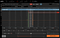Screenshot of Tastyworks (photo by author)

I decided to simulate it on the 122.5 Put, with 37 days to go. You can see at the bottom left hand corner the P50 which currently stands at 89%. We’ll try to match that.

# 1. Simulating Stock Price

As they explained in their video, the first thing they did was run a Monte Carlo simulation on the stock price. We’ll use the standard Geometric Brownian Motion model to simulate our stock price.

I pulled Apple’s stock price from Tiingo, this free website that gives better data than Yahoo and then calculated the log daily returns. Plotting it out, you get this: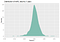AAPL returns (graph by author)

Which looks fine. Next, in order to perform GBM calculations, we have to find the drift (the mean) and the sigma (the standard deviation). I’m not going to go into detail about GBM calculations since the focus of this article is the P50 but you can find more of it here.

I got the gbm_vector calculation from here so credits to RobotWealth for that. I then set it up for our particular Put (which has 37 days to go), with 1000 simulations (as per the Tastyworks video).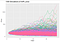AAPL price simulation (graph by author)

And that’s done! So we’ve completed the first part, which is the simulation of the stock price.

# 2. Simulating Put Price

Now that we had the stock price, all we had to do was calculate the Put price for each simulated price path. I wrote a function to calculate the Put price based on the BSM. Next, I created an empty matrix to hold the price of the Puts. And ran a nested loop to take into account (a) the 1000 simulations and (b) the number of days we were simulating for.

Once I had the matrix of the Put price, I converted it to a dataframe and then plotted it!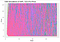AAPL put price simulation (graph by author)

I intentionally limited the “zoom” to only up to 25 as the put started at ~\$1.70 and we wanted to see when it would hit P50 (which is \$0.85) so it didn’t make sense to see the puts that had gone up to the \$100s. Of course, this graph was still incredibly messy so I just selected 10 random ones to make it look cleaner and limited the y-axis.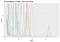AAPL put price simulation (graph by author)

As you can see, some did indeed dip below the \$0.85 mark. All we had to do now was quantify how many had dipped below that mark.

# Calculating P50

I first filtered the rows that had prices of less than 0.85. I just wanted to find out how many simulations had at least one value below 0.85 so I grouped it by simulation number and then kept only the head of each group.

Comparing our value of 86% to Tastywork’s, we see that theirs is 89%! So not too far off!

# Conclusion

Of course, this could easily be adapted to P70 if you wished or P20, just by changing the filtered price. Additionally, we could also extend it to spreads by calculating the individual prices of each leg and then adding the prices up. With that said, that’s about it for this article and thanks for reading! Let me know if you spot any errors or have any comments.

Originally published at zachlim98.github.io/me

Hi! I’m learning to explore data and think about personal finance (not always in that order)

## More from Zachary Lim

Hi! I’m learning to explore data and think about personal finance (not always in that order)